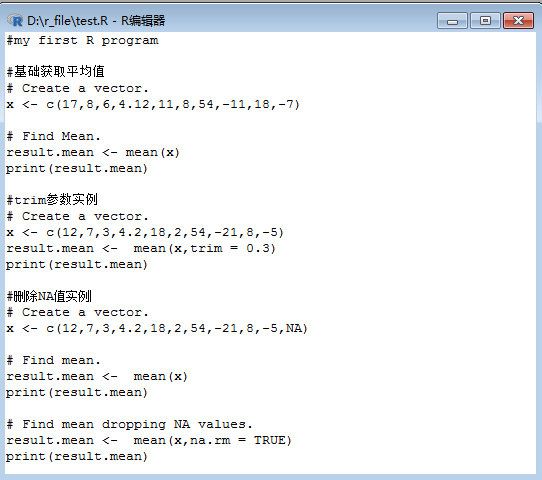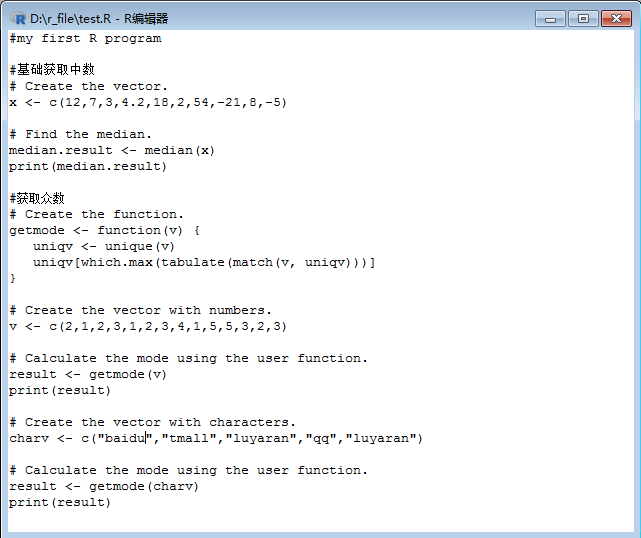• 平均值与中位数【难度:1级】: 答案1: from numpy import mean, median def mean_vs_median(numbers): if mean(numbers) > median(numbers): return 'mean' elif mean(numbers) < median(numbers): ...
平均值与中位数【难度:1级】:
答案1:
from numpy import mean, median

def mean_vs_median(numbers):
if mean(numbers) > median(numbers):
return 'mean'
elif mean(numbers) < median(numbers):
return 'median'
else:
return 'same'​


答案2:
import numpy as np

def mean_vs_median(numbers):
return {-1: "median", 0: "same", 1: "mean"}.get (np.sign(np.mean(numbers) - np.median(numbers)))
​


答案3:
def mean_vs_median(numbers):
mean = sum(numbers)/len(numbers)
med = sorted(numbers)[len(numbers)//2]
return 'mean' if mean > med else 'median' if med > mean else 'same'
​


答案4:
from numpy import mean,median

def mean_vs_median(N):
MEAN = int(mean(N))
MEDIAN = int(median(N))
return 'mean' if MEAN>MEDIAN else 'median' if MEDIAN>MEAN else 'same'
​


答案5:
import numpy as np

def mean_vs_median(numbers):
mean_v = np.mean(numbers)
median_v = np.median(numbers)
if mean_v < median_v:
return 'median'
elif mean_v > median_v:
return 'mean'
else:
return 'same'
​


答案6:
def mean_vs_median(numbers):
median = sorted(numbers)[len(numbers)//2]
mean = float(sum(numbers)) / len(numbers)

return 'mean' if mean > median else 'median' if median > mean else 'same'​


答案7:
def mean_vs_median(numbers):
numbers.sort()
n = len(numbers)
mean = float(sum(numbers)) / n
median = (numbers[n / 2] + numbers[~n / 2]) / 2.
return ['same', 'mean', 'median'][cmp(mean, median)]​


答案8:
def mean_vs_median(numbers):
mean = sum(numbers) / float(len(numbers))
median = sorted(numbers)[len(numbers) / 2]
return "mean" if mean > median else "median" if median > mean else "same"​


答案9:
from statistics import mean, median
def mean_vs_median(numbers):
avg, med = mean(numbers), median(numbers)
return ["mean", "same", "median"][avg == med or avg < med and 2]
​


答案10:
mean_vs_median=lambda n:'mean'if sum(n)/len(n)>n[int(len(n)/2)]else'same'if sum(n)/len(n)==n[int(len(n)/2)+1]else"median"​




展开全文python编程练习 基础 数组 数据类型
• R语言平均值中位数和众数 R中的统计分析通过使用许多内置函数来执行的。这些函数大部分是R基础包的一部分。这些函数将R向量参数一起作为输入，并在执行计算后给出结果。 我们在本章中讨论的是如何求平均值，...


R语言平均值，中位数和众数

R中的统计分析通过使用许多内置函数来执行的。这些函数大部分是R基础包的一部分。这些函数将R向量与参数一起作为输入，并在执行计算后给出结果。我们在本章中讨论的是如何求平均值，中位数和众数。下面将分别一个个演示和讲解 -

1.平均值

平均值是通过取数值的总和并除以数据序列中的值的数量来计算。函数mean()用于在R中计算平均值。

语法

R中计算平均值的基本语法是 -
mean(x, trim = 0, na.rm = FALSE, ...)
R
以下是使用的参数的描述 -
x -
是输入向量。
trim -
用于从排序的向量的两端删除一些观测值。
na.rm -
用于从输入向量中删除缺少的值。
示例
# Create a vector.
x <- c(17,8,6,4.12,11,8,54,-11,18,-7)
# Find Mean.
result.mean <- mean(x)
print(result.mean)
R
当我们执行上述代码时，会产生以下结果 -
 10.812
Shell

1.1.应用修剪选项

当提供trim参数时，向量中的值进行排序，然后从计算平均值中删除所需数量的观察值。

例如，当trim = 0.3时，每一端的3个值将从计算中删除以找到均值。

在这种情况下，排序的向量为(-21，-5,2,3,42,7,8,12,18,54)，从用于计算平均值的向量中从左边删除：(-21，-5,2)和从右边删除：(12,18,54)这几个值。
# Create a vector.
x <- c(12,7,3,4.2,18,2,54,-21,8,-5)
# Find Mean.
result.mean <-  mean(x,trim =
0.3)
print(result.mean)
R

当我们执行上述代码时，会产生以下结果 -
 5.55
Shell

1.2.应用NA选项

如果缺少值，则平均函数返回NA。要从计算中删除缺少的值，请使用na.rm = TRUE。 这意味着删除NA值。参考以下示例代码
-
# Create a vector.
x <- c(12,7,3,4.2,18,2,54,-21,8,-5,NA)
# Find mean.
result.mean <-  mean(x)
print(result.mean)
# Find mean dropping NA values.
result.mean <-  mean(x,na.rm =
TRUE)
print(result.mean)
R
当我们执行上述代码时，会产生以下结果 -
 NA
 8.22
Shell

2.中位数

数据系列中的中间值被称为中位数。R中使用median()函数来计算中位数。

语法

R中计算位数的基本语法是 -
median(x, na.rm = FALSE)
R

以下是使用的参数的描述 -
x -
是输入向量。
na.rm -
用于从输入向量中删除缺少的值。

示例
# Create the vector.
x <- c(12,7,3,4.2,18,2,54,-21,8,-5)
# Find the median.
median.result <- median(x)
print(median.result)
R
当我们执行上述代码时，会产生以下结果 -
 5.6
Shell

3.众数

众数是指给定的一组数据集合中出现次数最多的值。不同于平均值和中位数，众数可以同时具有数字和字符数据。

R没有标准的内置函数来计算众数。因此，我们将创建一个用户自定义函数来计算R中的数据集的众数。该函数将向量作为输入，并将众数值作为输出。

示例
# Create the function.
getmode <- function(v) {
uniqv <-
unique(v)

uniqv[which.max(tabulate(match(v, uniqv)))]
}
# Create the vector with numbers.
v <- c(2,1,2,3,1,2,3,4,1,5,5,3,2,3)
# Calculate the mode using the user function.
result <- getmode(v)
print(result)
# Create the vector with characters.
charv <-
c("baidu.com","tmall.com","yiibai.com","qq.com","yiibai.com")
# Calculate the mode using the user function.
result <- getmode(charv)
print(result)
R

当我们执行上述代码时，会产生以下结果 -
 2
 "yiibai.com"

转载于:https://www.cnblogs.com/amengduo/p/9586549.html
展开全文• ## 中位数和平均值

万次阅读 2018-09-27 23:09:44
部分数据的变动对中位数没有影响，当一组数据中的个别数据变动较大时，常用它来描述这组数据的集中趋势。 3）众数也是数据的一种代表数，反映了一组数据的集中程度．日常生活中诸如“最佳”、“最受欢迎”、“最...
区别联系

1）平均数是通过计算得到的，因此它会因每一个数据的变化而变化。

2）中位数是通过排序得到的，它不受最大、最小两个极端数值的影响。部分数据的变动对中位数没有影响，当一组数据中的个别数据变动较大时，常用它来描述这组数据的集中趋势。

3）众数也是数据的一种代表数，反映了一组数据的集中程度．日常生活中诸如“最佳”、“最受欢迎”、“最满意”等，都与众数有关系，它反映了一种最普遍的倾向。

优缺点：

平均数：需要全组所有数据来计算；易受数据中极端数值的影响。中位数：仅需把数据按顺序排列后即可确定；不易受数据中极端数值的影响。众数：通过计数得到；不易受数据中极端数值的影响。

对于有限的数集，可以通过把所有观察值高低排序后找出正中间的一个作为中位数。如果观察值有偶数个，通常取最中间的两个数值的平均数作为中位数。

中位数：也就是选取中间的数，是一种衡量集中趋势的方法。

例1

找出这组数据：23、29、20、32、23、21、33、25 的中位数。

解：

首先将该组数据进行排列（这里按从小到大的顺序），得到：

20、21、23、23、25、29、32、33

因为该组数据一共由8个数据组成，即n为偶数，故按中位数的计算方法，得到中位数，即第四个数和第五个数的平均数。

例2

找出这组数据：10、20、 20、 20、 30的中位数。

解：

首先将该组数据进行排列（这里按从小到大的顺序），得到：

10、 20、 20、 20、 30

因为该组数据一共由5个数据组成，即n为奇数，故按中位数的计算方法，得到中位数为20，即第3个数。
展开全文• R的统计分析通过使用...平均值是通过取数值的总和并除以数据序列的值的数量来计算，函数mean()用于在R计算平均值，语法如下： mean(x, trim = 0, na.rm = FALSE, ...) 参数描述如下： x - 是输入向量。 t...
R中的统计分析通过使用许多内置函数来执行的，这些函数大部分是R基础包的一部分，并且它们将R向量与参数一起作为输入，并在执行计算后给出结果。

先来看如何求平均值。

平均值是通过取数值的总和并除以数据序列中的值的数量来计算，函数mean()用于在R中计算平均值，语法如下：

mean(x, trim = 0, na.rm = FALSE, ...)

参数描述如下：

x - 是输入向量。
trim - 用于从排序的向量的两端删除一些观测值。
na.rm - 用于从输入向量中删除缺少的值。
当我们提供trim参数时，向量中的值进行排序，然后从计算平均值中删除所需数量的观察值，例如，当trim = 0.3时，每一端的3个值将从计算中删除以找到均值。在这种情况下，排序的向量为(-21，-5,2,3,42,7,8,12,18,54)，从用于计算平均值的向量中从左边删除：(-21，-5,2)和从右边删除：(12,18,54)这几个值。

如果缺少值，则平均函数返回NA，我们如果要从计算中删除缺少的值，可以使用na.rm = TRUE， 这意味着删除NA值。

好啦，来综合看下实例：输出结果为：数据系列中的中间值被称为中位数，在R中使用median()函数来计算中位数，语法如下：

median(x, na.rm = FALSE)

参数描述如下：

x - 是输入向量。
na.rm - 用于从输入向量中删除缺少的值。
众数是指给定的一组数据集合中出现次数最多的值，不同于平均值和中位数，众数可以同时具有数字和字符数据。R没有标准的内置函数来计算众数，因此，我们将创建一个用户自定义函数来计算R中的数据集的众数。该函数将向量作为输入，并将众数值作为输出，来分别看下实例：输出结果为：好啦，本次记录就到这里了。

如果感觉不错的话，请多多点赞支持哦。。。
展开全文R语言
• 近期整理总结了2014-2018杜克数学竞赛(DMM)个人赛(Individual Round)的历年真题，看到了两道关于统计量(平均与中位数)的赛题，还挺有趣的。因为这两个统计量比较简单所以就不过多介绍了，直接分享赛题了。题意：把...
• const sampleStats = function(count) { let min = Infinity; let max = -Infinity; let sum = 0; let totalCount = 0; let modCount = 0; let mod; for (let i = 0; i < count.length;......js
• 用二进制求两平均值的话，大致思路在于找出二进制种对应相同的和对应不同的，分别求解平均值再求和。相同平均值即为相同的本身，不同平均值还需除以2，即二进制右移一。 取7和6为例： 7&6...
• 我们在本章中讨论的是如何求平均值中位数和众数。下面将分别一个个演示和讲解 -1.平均值平均值是通过取数值的总和并除以数据序列中的值的数量来计算。函数mean()用于在R中计算平均值。语法R中计算平均值的基本语法...mysql 众数
• 课上老师用的课本是《MapReduce编程设计模式》，里面第一章就介绍了Wordcount ，接下来就是最大值最小值平均值标准差，其数据来源于Stack Overflow网站上的评论内容，包括评论时间、评论用户ID，评论文本。...
• 如果各要素存在z属性，则该工具还会计算第三维的中心:说明：平均中心工具会创建一个新的点要素类，要素类的每个要素表示一个平均中心(如果您指定了案例分组字段，则每个案例都会对应一个平均中心)。X 和 Y 平均...
• 今天在CSDN上看了一个求两个平均值的算法：Avg = (ValueA & ValueB) + (ValueA ^ ValueB) >>1，这种方法避免了应用Avg=（ValueA+ValueB）/2时，ValueA+ValueB造成的溢出。  每个二进都可以分解为各个...C++ 算法
• 在他看来，通过较高的水价收费，能够有效遏制生活和工业的水资源浪费：“目前用水成本在家庭开支占比小，没有家庭因为交水费而交穷的。 感慨下物价飞涨，政策乱行的同时，老陆评论：当前在新加坡每个月水电费...
• 中位数：像一条分界线，将数据分成前半部分和后半部分，因此用来代表一组数据的“一般水平”。 众数：反映了出现次数最多的数据，用来代表一组数据的“多数水平”。 平均数：每一个数据都有关,其中任何数据的...
• 将数据排序后，位置在最中间的数值。即将数据分成两部分，一部分大于该数值，一部分...中位数的作用算术平均数相近，也是作为所研究数据的代表。在一个等差数列或一个正态分布数列中，中位数就等于算术平均数...
• 在初中数学课本中，我们学习了平均数，但是平均与中位数、众数有是关系呐，下面我就为大家总结一下：平均数：是指在一组数据中所有数据之和再除以数据的个数。平均数是表示一组数据集中趋势的量数，它是反映数据...
• 将数据排序后，位置在最中间的数值。即将数据分成两部分，一部分大于该数值，一部分...中位数的作用算术平均数相近，也是作为所研究数据的代表。在一个等差数列或一个正态分布数列中，中位数就等于算术平均数...
• python - pandas DataFrame：用平均列...这个问题这个问题非常相似：numpy数组：用列的平均值替换nan值，但不幸的是，给出的解决方案不适用于pandas DataFrame。piokuc asked 2019-07-29T20:10:32Z8个解决方案1...
• 中位数的作用算术平均数相近，也是作为所研究数据的代表。在一个等差数列或一个正态分布数列中，中位数就等于算术平均数。 　在数列中出现了极端变量的情况下，用中位数作为代表要...
• 课上老师用的课本是《MapReduce编程设计模式》，里面第一章就介绍了Wordcount ，接下来就是最大值最小值平均值标准差，其数据来源于Stack Overflow网站上的评论内容，包括评论时间、评论用户ID，评论文本。...
• 一般方法：(x + y) / 2存在问题：当两个比较大时，如果两个的和大于了机器位数能够表示的最大，可能会存在数据溢出的情况改进：采用运算方法(x &amp; y) + ((x ^ y) &gt;&gt; 1)(x &amp; y)...
• Average = 平均值”顺序输出和与平均值，其中平均值精确到小数点后一。 输入样例: 1 2 3 4 输出样例: Sum = 10; Average = 2.5 import java.util.Scanner; public class Main{ public static void main(String...java
• 可用numpy.mean函数计算中位数： 数字从小到大排列的数列中位于中间位置的那个数，如果数列个数为奇数位则中位数位于(n+1)/2, 如果为偶数位则为n/2n/2+1的两个数的平均值，可以用numpy.median函数计算众数：数列中...
• 说明：这是武汉理工大学计算机学院【算法设计与分析】课程的第一次实验第一题：分治法与中位数问题 >>点击查看WUTer计算机专业实验汇总 谨记：纸上得来终觉浅，绝知此事要躬行。 一、问题描述 输入任意一...分治法
• ## 中位数

千次阅读 2013-03-19 10:23:57
中位数（Medians）统计学名词，是指将统计总体当中的各个变量按大小顺序排列起来，形成一个数列，处于变量... 中位数的作用算术平均数相近，也是作为所研究数据的代表。在一个等差数列或一个正态分布数列中，中
• python基础练习题:与平均中位数【难度:1级】: 你的目标是实现它接受一个整数* 奇数长度* 数组矩阵和返回以下中的一个的方法meanVsMedian: “的意思是” - 如果* ** 平均价值比** ** 中值较大 “平均” - 万一* ** ...python编程练习 数据类型...